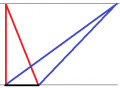# Find angles and sides of a triangle; given height and area

#### ace2015

Joined Oct 24, 2017
15
Find angles and sides of a triangle:

Given that the height = 20m and the area = 20m^2

What formulas should I use to calculate the angles and the sides?

Best regards

#### atferrari

Joined Jan 6, 2004
4,473
Find angles and sides of a triangle:

Given that the height = 20m and the area = 20m^2

What formulas should I use to calculate the angles and the sides?

Best regards
Draw what you believe is the figure representing the problem and post it here.

Formulas with no reasoning are like recipes.

•ace2015

#### LesJones

Joined Jan 8, 2017
3,571
Could you answer the question if it was a rectangle rather than a triangle. That is the only way I can think of giving you a hint on how to do it without giving the answer.

Les.

•ace2015

#### MrChips

Joined Oct 2, 2009
24,620
Is there a unique solution?

If there is a unique solution (i.e. a single solution) then you are missing a vital piece of information.

•ace2015

#### ace2015

Joined Oct 24, 2017
15
Its ok I figured it out. Thanks for your input.

#### atferrari

Joined Jan 6, 2004
4,473

#### ace2015

Joined Oct 24, 2017
15

#### WBahn

Joined Mar 31, 2012
26,398
Are you sure?

Consider the following construction:

1) Draw a horizontal line.
2) Draw 20 m vertical line upward starting from some point on the horizontal line.
3) Pick a random point on the horizontal line and draw a line segment from that point to the top of the vertical line.
4) Move along the horizontal line to the right until the area of the triangle formed by the top of the vertical line, the randomly picked point, and the current location is equal to 20 m².

Is it your assertion that the resulting triangle must look like your solution?

#### shteii01

Joined Feb 19, 2010
4,644
um... you said area is 20 m^2 and height is 20 m. Now, magically, area is 18 and height is 18.

•absf

#### Dodgydave

Joined Jun 22, 2012
10,088
Find angles and sides of a triangle:

Given that the height = 20m and the area = 20m^2

What formulas should I use to calculate the angles and the sides?

Best regards
Area = 1/2 B x H, so 20 =1/2 B x 20

Transpose the formula for B..

#### LesJones

Joined Jan 8, 2017
3,571
Hi Dave,
II was hoping the question I asked in post #3 was a good enough hint to the method you have just suggested.
Les.

#### WBahn

Joined Mar 31, 2012
26,398
Area = 1/2 B x H, so 20 =1/2 B x 20

Transpose the formula for B..
How does that help address the TS's problem?

For instance, consider the following two triangles which have the same height and the same area.Yes, they thus have the same base. So what?

They clearly do NOT have the same angles or the same sides, which is what the TS is supposed to find.